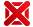Stochastic Control And Mathematical Modeling - 9780521195034

Categorias: Idiomas | Inglês

Stochastic Control And Mathematical Modeling Morimoto, Hiroaki Cambridge Usa
Avaliações

Por: R\$ 928,27

em até 4x sem juros no cartãoProduto indisponívelEste produto está temporariamente indisponível.
Nós avisaremos quando ele chegar.

Sinopse

This is a concise and elementary introduction to stochastic control and mathematical modelling. This book is designed for researchers in stochastic control theory studying its application in mathematical economics and those in economics who are interested in mathematical theory in control. It is also a good guide for graduate students studying applied mathematics, mathematical economics, and non-linear PDE theory. Contents include the basics of analysis and probability, the theory of stochastic differential equations, variational problems, problems in optimal consumption and in optimal stopping, optimal pollution control, and solving the Hamilton-Jacobi-Bellman (HJB) equation with boundary conditions. Major mathematical prerequisites are contained in the preliminary chapters or in the appendix so that readers can proceed without referring to other materials.

Detalhes do produto

 Peso: 0,61 kg Número de páginas: 340 Ano de edição: 2010 ISBN 10: 0521195039 ISBN 13: 9780521195034 Altura: 3 Largura: 16 Comprimento: 24 Idioma : Inglês Tipo de produto : Livro Assuntos : Ciências Exatas Assuntos : Matemática